#### You may also like### Chocolate

There are three tables in a room with blocks of chocolate on each. Where would be the best place for each child in the class to sit if they came in one at a time?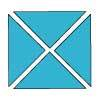### Four Triangles Puzzle

Cut four triangles from a square as shown in the picture. How many different shapes can you make by fitting the four triangles back together?### Cut it Out

Can you dissect an equilateral triangle into 6 smaller ones? What number of smaller equilateral triangles is it NOT possible to dissect a larger equilateral triangle into?

# Fractions in a Box

##### Age 7 to 11Challenge Level

We have a game which has a number of discs in seven different colours. These are kept in a flat square box with a square hole for each disc. There are $10$ holes in each row and $10$ in each column. So, there would be $100$ discs altogether, except that there is a square booklet which is kept in a corner of the box in place of some of the holes.

We haven't drawn a grid to show all the holes because that would give the answer away!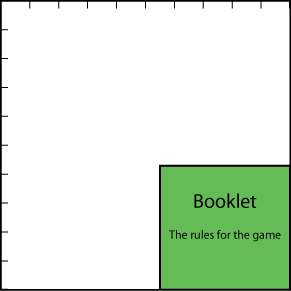There is a different number of discs of each of the seven colours.

Half ($\frac{1}{2}$ ) of the discs are red, $\frac{1}{4}$ are black and $\frac{1}{12}$ are blue.One complete row (of $10$ holes) of the box is filled with all the blue and green discs.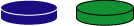One of the shortened rows (that is where the booklet is) is exactly filled with all the orange discs.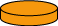Two of the shortened rows are filled with some of the red discs and the rest of the red discs exactly fill a number of complete rows (of $10$) in the box.

There is just one white disc and all the rest are yellow.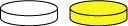How many discs are there altogether?
What fraction of them are orange?
What fraction are green? Yellow? White?